# Exercise E7.2 Triangles NCERT Solutions Class 9

## Chapter 7 Ex.7.2 Question 1

In an isosceles triangle $$ABC$$, with

$$AB = AC$$, the bisectors of $$\angle B$$ and $$\angle C$$

intersect each other at $$O$$. Join $$A$$ to $$O$$.

Show that:

(i) $$OB = OC$$

(ii) $$AO$$ bisects $$\angle A$$

### Solution

What is Known?

Triangle $$ABC$$ is isosceles in which $$AB=AC$$ also $$OB$$ and $$OC$$ are bisectors of angle $$B$$ and angle $$C$$

To prove:

(i) OB = OC

(ii) AO bisects ∠A

Reasoning:

As OB and OC are bisectors of angle B and angle C means half of angle B will be equal to half of angle c which will help us to conclude OB is equal to OC. Now We can show two triangles OAB and OAC congruent by using SSS congruency rule and then we can say corresponding parts of congruent triangles will be equal.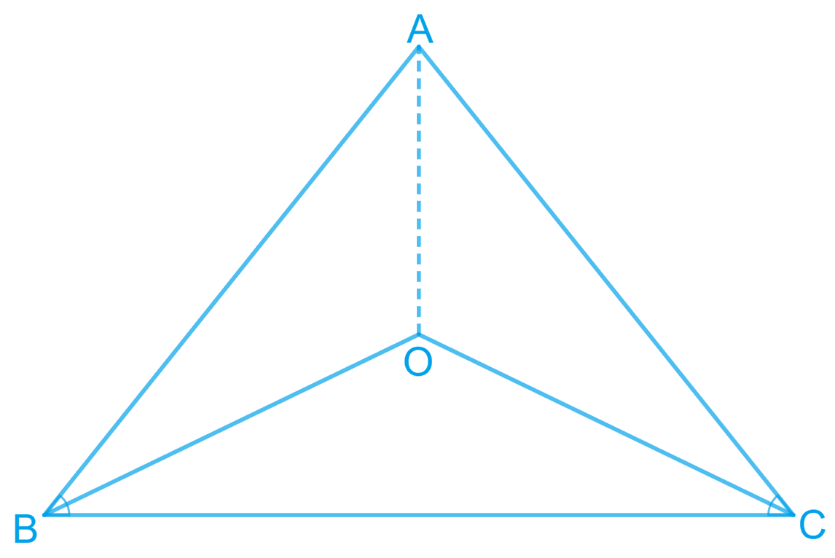Steps:

(i) It is given that in triangle $$ABC, AB = AC$$

\begin{align}& \angle ACB = \angle ABC \\&\left[ \begin{array} & \text{Angles opposite to equal } \\ \text{sides of a triangle are equal} \\ \end{array} \right]\\\\&\frac { 1 } { 2 } \angle ACB = \frac { 1 } { 2 } \angle ABC\end{align}

\begin{align} & \therefore OB=OC \\ & \left[ \begin{array} & \text{Sides}\,\,\text{opposite}\,\text{to}\,\text{equal}\,\text{angles}\, \\ \text{of}\,\text{a}\,\text{triangle}\,\text{are}\,\text{also}\,\text{equal} \\ \end{array} \right] \\ \end{align}

\begin{align} \rm (ii)\;\;&\text{In } \Delta OAB \text{ and } \Delta OAC, \\ & AO = AO\; (\text{Common}) \\ & AB = AC \;(\text{Given}) \\ & OB = OC\; (\text{Proved above}) \\ &\therefore \Delta OAB \cong \Delta OAC \\&\qquad(\text{By SSS congruence rule}) \end{align}

$\angle BAO = \angle CAO\;(CPCT)\\ \therefore AO \text{ bisects } \angle A$

## Chapter 7 Ex.7.2 Question 2

In $$\Delta ABC, AD$$ is the perpendicular bisector of $$BC$$ (see the given figure). Show that $$\Delta ABC$$ is an isosceles triangle in which $$AB = AC$$.

### Solution

What is Known?

$$AD$$ is perpendicular bisector of $$BC$$ means and $$BD=DC$$

To prove:

$$\Delta ABC$$ is an isosceles triangle in which $$AB = AC.$$

Reasoning:

We can show two triangles ADB and ADC congruent by using SAS congruency rule and then we can say corresponding parts of congruent triangles will be equal.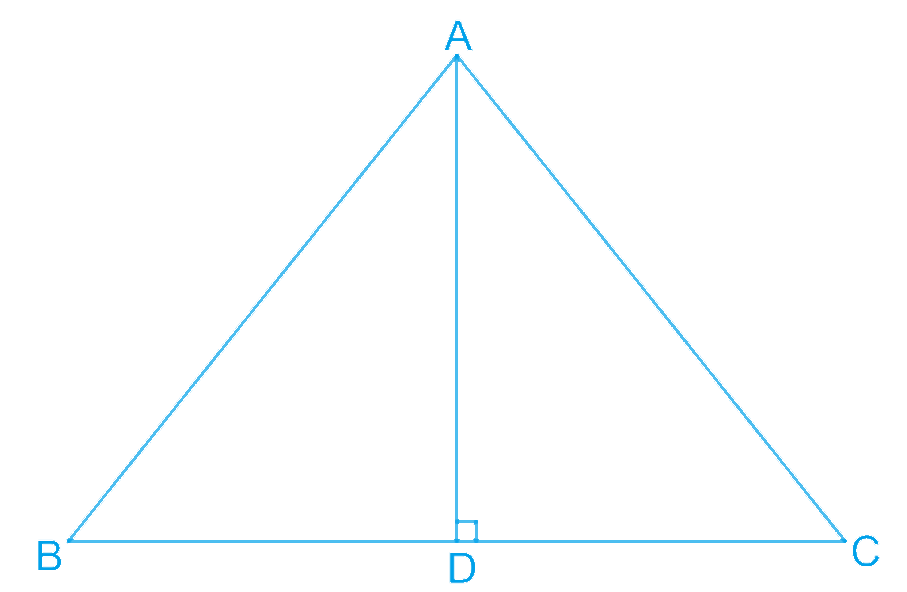Steps:

In $$\Delta ADC\text{ and }\Delta ADB,$$

\begin{align}&AD=AD (Common)\\&\angle ADC=\angle ADB\ (\text{Each }\!\!~\!\!\text{ }{{90}^{{}^\circ }})\\&CD=BD\\&\left( \begin{array} & \text{AD is the perpendicular } \\ \text{bisector of BC} \\ \end{array} \right)\\\\&\therefore \Delta ADC\cong \Delta ADB \\&\text{(By SAS congruence rule)}\\\\&\therefore AB=AC\,\,\,(\text{By }CPCT)\!\!~\!\!\end{align}

Therefore, $$ABC$$ is an isosceles triangle in which $$AB = AC$$.

## Chapter 7 Ex.7.2 Question 3

$$ABC$$ is an isosceles triangle in which altitudes $$BE$$ and $$CF$$ are drawn to equal sides $$AC$$ and $$AB$$ respectively (see the given figure). Show that these altitudes are equal.

### Solution

What is known?

Sides $$AB=AC,$$

$$BE\bot AC\ \text{and CF}\bot \text{AB}$$

To prove:

Altitudes $$BE$$ and $$CF$$ are equal or $$BE = CF$$

Reasoning:

We can show two triangles $$ABE$$ and $$ACF$$ congruent by using AAS congruency rule and then we can say corresponding parts of congruent triangles will be equal.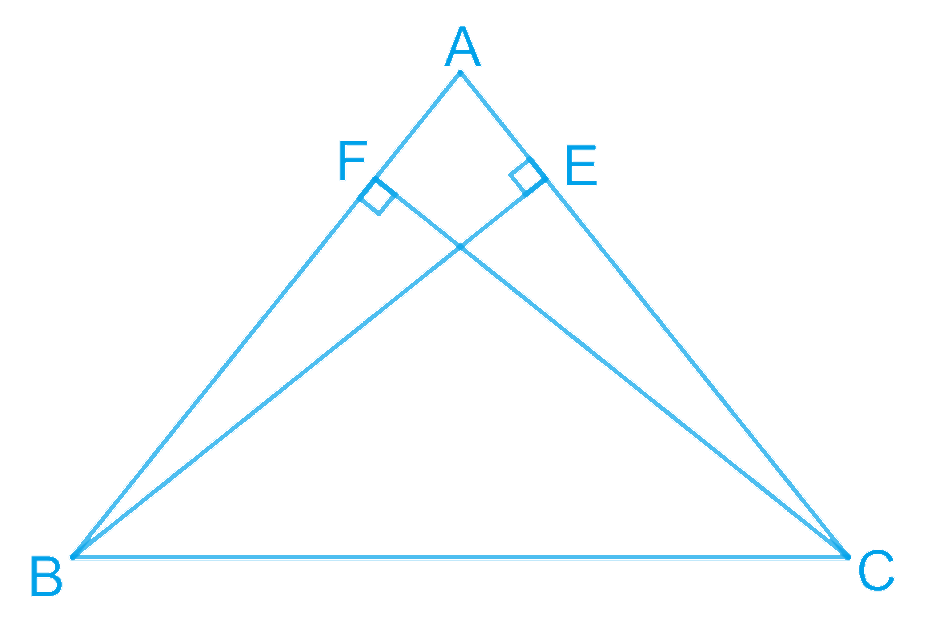Steps:

$$\text{In } \Delta AEB \text{ and } \Delta AFC,$$

\begin{align}& \angle AEB = \angle AFC \;(\text{Each }90^{\circ}) \\ & \angle A = \angle A\; (\text{Common angle}) \\ & AB = AC\; (\text{Given}) \\ & \therefore \Delta AEB \cong \Delta AFC \\& \text{(By AAS congruence rule)} \end{align}

$$\therefore BE = CF$$ (By $$CPCT$$ )

## Chapter 7 Ex.7.2 Question 4

$$ABC$$ is a triangle in which altitudes $$BE$$ and $$CF$$ to sides $$AC$$ and $$AB$$ are equal (see the given figure).

Show that:

(i) $$\ \Delta ABE \cong \Delta ACF$$

(ii) $$AB=AC,$$ i.e., ABC is an isosceles triangle.

### Solution

What is Known?

Altitudes,$$\text{BE}=\text{CF},\text{ BE}\bot \text{AC}\ \text{and CF}\bot \text{AB}$$

To prove:

(i) $$\ \Delta ABE \cong \Delta ACF$$
(ii) $$AB=AC,$$ i.e., ABC is an isosceles triangle.

Reasoning:

We can show two triangles $$ABE$$ and $$ACF$$ congruent by using AAS congruency rule and then we can say corresponding parts of congruent triangles will be equal.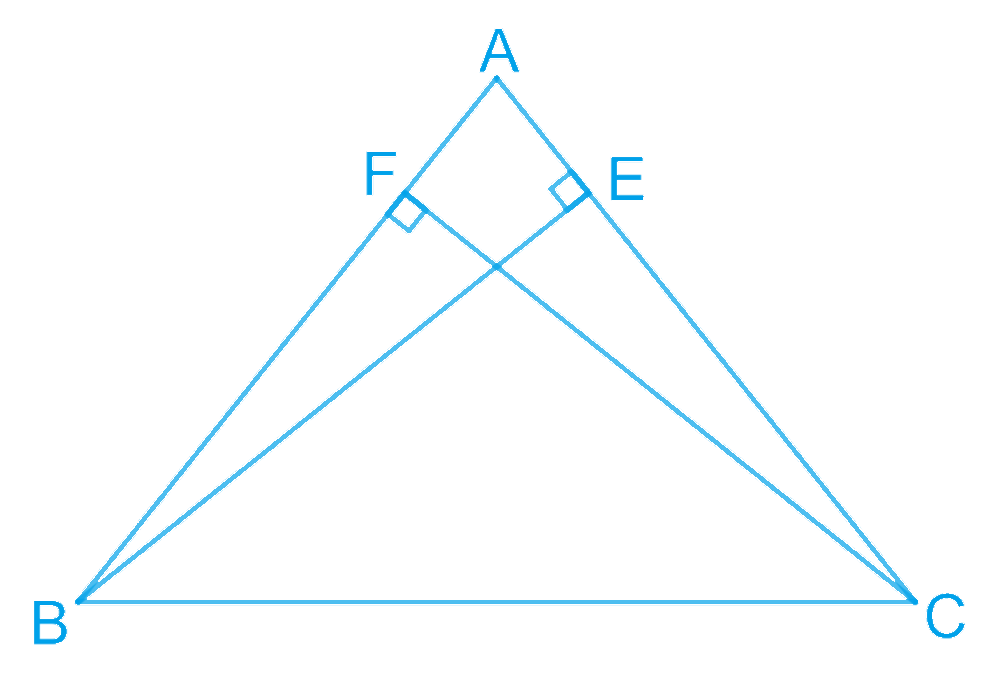Steps:

(i) In $$\Delta ABE\text{ and }\Delta ACF,$$

\begin{align}& \angle AEB \text{ and }\angle AFC \;(\text{Each} 90^{\circ}) \\ \angle A&=\angle A \;(\text{Common angle}) \\ BE&=CF \; (\text{Given}) \\ \therefore \Delta ABE &\cong \Delta ACF \\&(\text{By AAS congruence rule}) \end{align}

(ii) It has already been proved that

\begin{align}&\Delta ABE \cong \Delta ACF, \\ &\therefore AB = AC \; (\text{By }CPCT) \\ \end{align}

## Chapter 7 Ex.7.2 Question 5

$$ABC$$ and $$DBC$$ are two isosceles triangles on the same base $$BC$$ (see the given figure). Show that $$∠ABD = ∠ACD.$$

### Solution

What is Known?

$$ABC$$ and $$DBC$$ are two isosceles triangles.

To prove:

$$\angle \text{ABD}=\angle \text{ACD}$$

Reasoning:

First of all, we can join point $$A$$ and $$D$$ then we can show two triangles $$ADB$$ and $$ADC$$ congruent by using SSS congruency rule after that we can say corresponding parts of congruent triangles will be equal.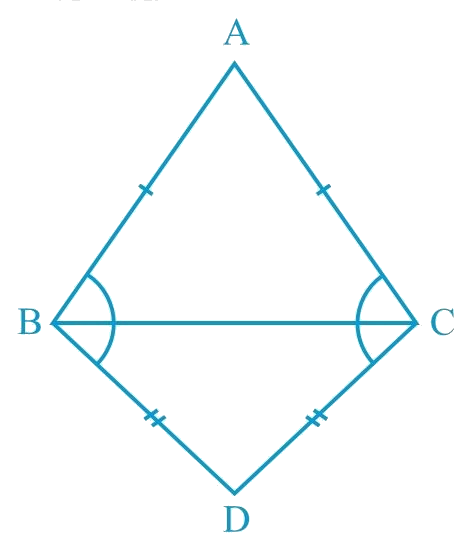Steps: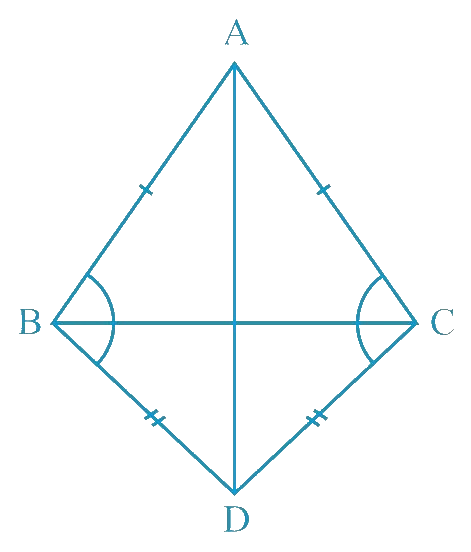Let us join $$AD.$$

In $$\triangle ABD \text { and } \Delta ACD,$$

\begin{align}&AB = AC\;( \text {Given} ) \\ &BD = CD( \text {Given} )\\ &AD = AD \; (\text{Common side}) \\& \therefore \Delta ABD \cong \Delta ACD \\&(\text{By SSS congruence rule}) \end{align}

\begin{align}&\therefore \Delta ABD = \Delta ACD \;(\text{By }CPCT) \end{align}

## Chapter 7 Ex.7.2 Question 6

$$\Delta ABC$$ is an isosceles triangle in which $$AB = AC$$. Side $$BA$$ is produced to $$D$$ such that $$AD = AB$$ (see the given figure).

Show that $$\angle BCD$$ is a right angle.

### Solution

What is Known?

Sides $$AB=AC$$ and $$AD=AB$$

To prove:

$$∠BCD$$ is a right angle.

Reasoning:

We can use the property angles opposite to equal sides are equal and then by the angle sum property in triangle $$BCD$$ we can show the required result.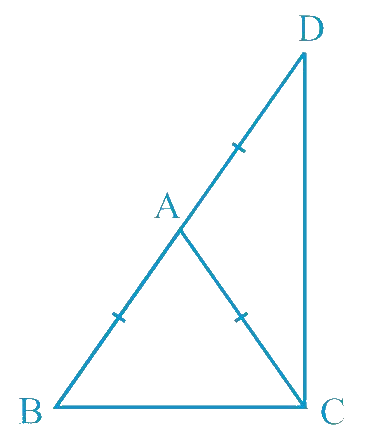Steps:

In $$\Delta ABC,$$

\begin{align} &\qquad\;\;\, AB=AC(\text{Given}) \\ &\therefore \angle ACB=\angle ABC \\ & \left( \begin{array}{I} \text{Angles opposite to equal sides } \\ \text{of a triangle are also equal}\text{.} \\ \end{array} \right) \\ \end{align}

In $$\Delta ACD,$$\begin{align} &\qquad\;\;\, AC=AD \\ &\therefore \angle ADC=\angle ACD \\ & \left( \begin{array}{I} \text{Angles opposite to equal sides } \\ \text{of a triangle are also equal}\text{.} \\ \end{array} \right) \\ \end{align}

In $$\Delta BCD,$$

\begin{align}& \angle ABC+\angle BCD+\angle ADC=180^{\circ} \\&(\text{Angle sum property of a }\text{triangle}) \\\\& \angle ACB\!+\!\!\angle ACB\!+\!\!\angle ACD\!+\!\!\angle ACD\!=\!180^{\circ} \\& 2(\angle ACB+\angle ACD)=180^{\circ} \\& 2(\angle BCD)=180^{\circ} \\& \therefore \angle BCD=90^{\circ} \end{align}

## Chapter 7 Ex.7.2 Question 7

$$ABC$$ is a right-angled triangle in which

$$\angle A = 90^{\circ}$$ and $$AB = AC$$. Find $$\angle B$$ and $$\angle C$$.

### Solution

What is Known?

$$ABC$$ is right-angled triangle and sides $$AB=AC$$

To Find:

Value of $$∠B$$ and $$∠C.$$

Reasoning:

We can use the property angles opposite to equal sides are equal and then by the angle sum property in triangle $$ABC$$ we can find the value of $$∠B$$ and $$∠C.$$

Steps: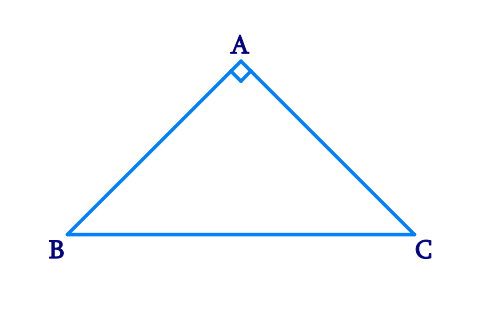It is given that

\begin{align} &\quad\, AB=AC\\ &\therefore \angle C=\angle B \\ & \left( \begin{array}{I} \text{Angles opposite to equal sides } \\ \text{of a triangle are also equal}\text{.} \\ \end{array} \right) \\ \end{align}

In $$\Delta ABC,$$

\begin{align} \angle A+\angle B+\angle C&=180^{\circ}\\ (\text{Angle sum property}&\text{ of a triangle}) \\\\ 90^{\circ}+\angle B+\angle C&=180^{\circ} \\ 90^{\circ}+\angle B+\angle B&= 180^{\circ} \\ 2\angle B&= 90^{\circ} \\ \angle B&=45^{\circ} \\ \therefore \angle B=\angle C&=45^{\circ} \\ \end{align}

## Chapter 7 Ex.7.2 Question 8

Show that the angles of an equilateral triangle are $$60^{\circ}$$ each.

### Solution

What is Known?

Triangle $$ABC$$ is an equilateral triangle.

To prove:

The angles of an equilateral triangle are $$60º$$ each.

Reasoning:

We can use the property angles opposite to equal sides are equal and then by the angle sum property in triangle $$ABC$$ we can show the value of each angle is $$60$$ degree.

Steps: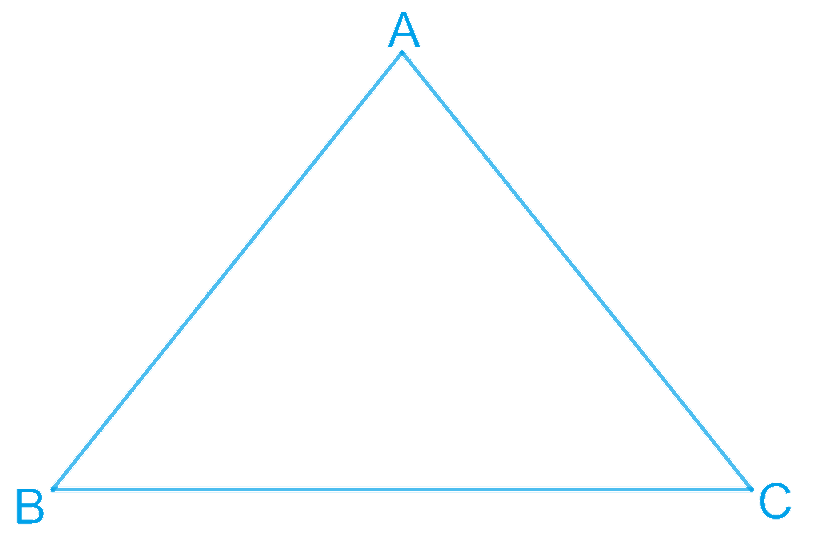Let us consider that $$ABC$$ is an equilateral triangle.

Therefore,

\begin{align} &AB=BC=AC \\ &AB=AC \\ \therefore \,&\angle C=\angle B \\ & \left( \begin{array}{I} \text{Angles opposite to equal sides } \\ \text{of a triangle are also equal}\text{.} \\ \end{array} \right) \\ \end{align}

Therefore, we obtain $$\angle A = \angle B = \angle C$$

In $$\Delta ABC,$$
\begin{align} \angle A+\angle B+\angle C&=180^{\circ} \\ \angle A+\angle A+\angle A&=180^{\circ} \\ 3\angle A&=180^{\circ} \\ \angle A&=60^{\circ} \\ \therefore \angle A=\angle B&=\angle C=60^{\circ} \\ \end{align}

Hence, in an equilateral triangle, all interior angles are of measure $$60^{\circ}$$.

Instant doubt clearing with Cuemath Advanced Math Program